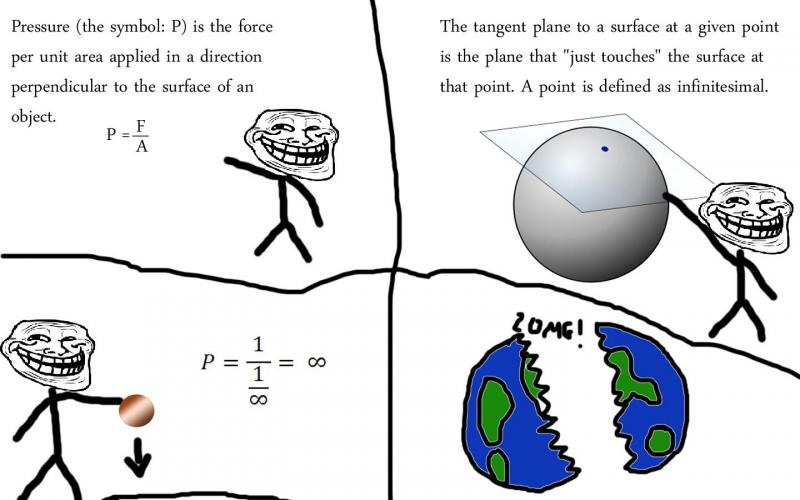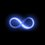# $\forall \exists \emptyset | \mathbf{R} \cup \mathbf{C}$Problem?Note by John M.
5 years, 12 months ago

This discussion board is a place to discuss our Daily Challenges and the math and science related to those challenges. Explanations are more than just a solution — they should explain the steps and thinking strategies that you used to obtain the solution. Comments should further the discussion of math and science.

When posting on Brilliant:

• Use the emojis to react to an explanation, whether you're congratulating a job well done , or just really confused .
• Ask specific questions about the challenge or the steps in somebody's explanation. Well-posed questions can add a lot to the discussion, but posting "I don't understand!" doesn't help anyone.
• Try to contribute something new to the discussion, whether it is an extension, generalization or other idea related to the challenge.

MarkdownAppears as
*italics* or _italics_ italics
**bold** or __bold__ bold
- bulleted- list
• bulleted
• list
1. numbered2. list
1. numbered
2. list
Note: you must add a full line of space before and after lists for them to show up correctly
paragraph 1paragraph 2

paragraph 1

paragraph 2

[example link](https://brilliant.org)example link
> This is a quote
This is a quote
    # I indented these lines
# 4 spaces, and now they show
# up as a code block.

print "hello world"
# I indented these lines
# 4 spaces, and now they show
# up as a code block.

print "hello world"
MathAppears as
Remember to wrap math in $$ ... $$ or $ ... $ to ensure proper formatting.
2 \times 3 $2 \times 3$
2^{34} $2^{34}$
a_{i-1} $a_{i-1}$
\frac{2}{3} $\frac{2}{3}$
\sqrt{2} $\sqrt{2}$
\sum_{i=1}^3 $\sum_{i=1}^3$
\sin \theta $\sin \theta$
\boxed{123} $\boxed{123}$

Sort by:

The analogy to this is that if you have a taut straight wire stretched between two fixed points, any force applied to the wire at about the center of it and at right angles to it will generate infinite tensile strain in the wire. Hence, universes can implode. See Calvin Lin

- 5 years, 12 months ago

Sup Michael!

What's a taut straight wire? Oh, and I think your TeX needs some FeXing

I'ma go check out that link real quick - oh wait where'd it go :O and what about the universe exploding and all that

- 5 years, 12 months ago

If the force by the plane on the sphere is also zero, then the expression for $P$ is $\frac{0}{0}=\boxed{1}$

Simple

- 5 years, 12 months ago

Yes, and $\pi=e$.

- 5 years, 12 months ago

I think we broke math

- 5 years, 12 months ago

Lactobacillus acidophilus bacteria are helpful in making yogurt.

- 5 years, 11 months ago